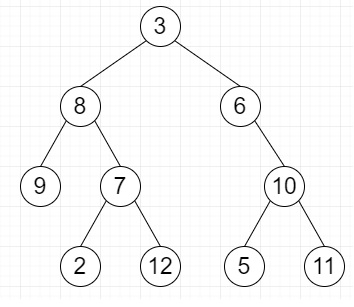# Find multiplication of sums of data of leaves at same levels in Python

Suppose we have a Binary Tree. We have to perform following operations −

• For each level, find sum of all leaves if there are leaves at this level. Otherwise ignore it.

• Find multiplication of all sums and return it.

So, if the input is likethen the output will be 270. First two levels have no leaves. Third level has single leaf 9. Last level has four leaves 2, 12, 5 and 11. So result is 9 * (2 + 12 + 5 + 11) = 270

To solve this, we will follow these steps −

• if root is null, then

• return 0

• res := 1

• que := a queue

• insert root at the end of que

• Do infinitely −

• no_of_nodes := size of que

• if no_of_nodes is same as 0, then

• come out from the loop

• sum_level := 0

• found_leaf := False

• while no_of_nodes > 0, do

• curr_node := first element of que

• if curr_node is leaf, then

• found_leaf := True

• sum_level := sum_level + curr_node.data

• delete first element from que

• if curr_node.left is not null, then

• insert curr_node.left at the end of que

• if curr_node.right is not null, then

• insert curr_node.right at the end of que

• no_of_nodes := no_of_nodes - 1

• if found_leaf is true, then

• res := res * sum_level

• return res

## Example

Let us see the following implementation to get better understanding −

Live Demo

class TreeNode:
def __init__(self, data):
self.data = data
self.left = self.right = None
def isLeaf(root) :
return (not root.left and not root.right)
def find_res(root) :
if (not root) :
return 0
res = 1
que = []
que.append(root)
while (True):
no_of_nodes = len(que)
if (no_of_nodes == 0) :
break
sum_level = 0
found_leaf = False
while (no_of_nodes > 0) :
curr_node = que
if (isLeaf(curr_node)) :
found_leaf = True
sum_level += curr_node.data
que.pop(0)
if (curr_node.left != None) :
que.append(curr_node.left)
if (curr_node.right != None) :
que.append(curr_node.right)
no_of_nodes -=1
if (found_leaf) :
res *= sum_level
return res
root = TreeNode(8)
root.left = TreeNode(8)
root.right = TreeNode(6)
root.left.right = TreeNode(7)
root.left.left = TreeNode(9)
root.left.right.left = TreeNode(2)
root.left.right.right = TreeNode(12)
root.right.right = TreeNode(10)
root.right.right.left = TreeNode(5)
root.right.right.right = TreeNode(11)
print(find_res(root))

## Input

root = TreeNode(8)
root.left = TreeNode(8)
root.right = TreeNode(6)
root.left.right = TreeNode(7)
root.left.left = TreeNode(9)
root.left.right.left = TreeNode(2)
root.left.right.right = TreeNode(12)
root.right.right = TreeNode(10)
root.right.right.left = TreeNode(5)
root.right.right.right = TreeNode(11)

## Output

270

Updated on: 19-Aug-2020

42 Views